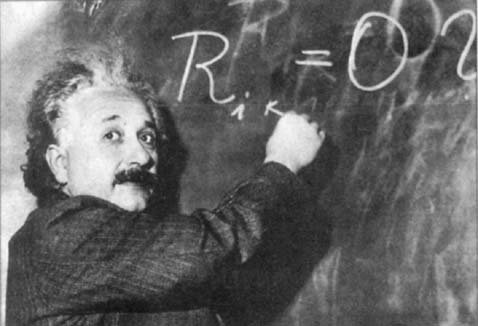# Mathematical Relativity — 2nd Semester 2011/2012

 Lecturer: José Natário Email: jnatar@math.tecnico.ulisboa.pt Office: Mathematics Building, 4th floor, room 4.29 Classes: Tuesdays and Fridays from 13:00 to 15:00. Office Hours: Tuesdays from 15:00 to 17:00.

## Syllabus

Examples: Minkowski space-time, Schwarzschild solution, Einstein, de Sitter, anti-de Sitter and Friedman-Lemaitre-Robertson-Walker universes; matching and Oppenheimer-Snyder collapse; Penrose diagrams.

Causality: time orientabiliy, chronological and causal past and future, domain of dependence; chronological, stably causal and globally hyperbolic spacetimes.

Singularities: Jacobi equation, conjugate points; energy conditions; existence of maximizing geodesics; Hawking and Penrose singularity theorems.

Cauchy Problem: wave equation; Cauchy problems with constraints; Gauss-Coddazzi relations and 3+1 decomposition of the Einstein equation; Choquet-Bruhat theorem; constraint equations for the initial data.

Positive Mass Theorem: Komar mass; Einstein-Hilbert action; Lagrangian and Hamiltonian formulation of the Einstein equations; mass of an asymptotically flat Riemannian 3-manifold; positive mass theorem; Penrose inequality.

Black Holes: Reissner-Nordstrom and Kerr solutions; cosmic censorship; area theorem; black hole thermodynamics.

Main

Secondary